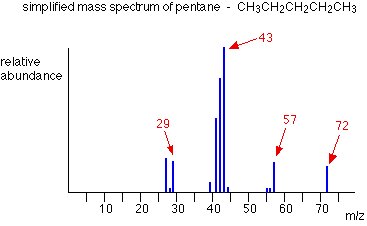# What would distinguish the mass spectrum of 2,2-dimethylpropane from the mass spectra of pentane and 2-methylbutane?

May 6, 2015

You examine the peaks at "m/z" = 59 ("M – CH"_3) and $\text{m/z} = 43$ (loss of ${\text{CH"_3"CH}}_{2}$).

• In 2,2-dimethylpentane, the base peak will be at $\text{m/z} = 59$
• In 2-methylbutane, both peaks will have comparable intensity
• In pentane, the base peak will be at $\text{m/z} = 43$

The structures of the three pentane isomers areMass spectroscopic fragmentation tends to occur at a branch.

2-2-Dimethylpropane (neopentane)

The base peak should be at $\text{m/z} = 57$. This corresponds to loss of a methyl group and formation of the stable t-butyl cation, ("CH"_3)_3"C"^+.2-Methylbutane (isobutane)

Here you have two possible fragmentations.

1. Loss of "CH"_3 ("M" -15).This will give a peak at $\text{m/z} = 57$, corresponding to ${\text{CH"_3"CH"^+"CH"_2"CH}}_{3}$.
2. Loss of "CH"_3"CH"_2 ("M" - 29). This will give a peak at $\text{m/z} = 43$, corresponding to ("CH"_3)_2"CH"^+.

The peaks at 43 and 29 should be stronger than those at 57 and 13, because the ethyl cation (29) is more stable than the methyl cation.

The base peak is probably $\text{m/z} = 43$.Pentane

Again, there are two possible fragmentations.

1. Loss of "CH"_3 ("M" -15).This will give a peak at $\text{m/z} = 57$, corresponding to ${\text{CH"_3"CH"_2"CH"_2"CH}}_{2}^{+}$.
2. Loss of "CH"_3"CH"_2 ("M"- 27). This will give a peak at $\text{m/z} = 45$, corresponding to ${\text{CH"_3"CH"_2"CH}}_{2}^{+}$.The peaks at 43 and 27 will be more intense that those at 57 and 15, because the second pair involves formation of the unstable methyl cation ${\text{CH}}_{3}^{+}$.